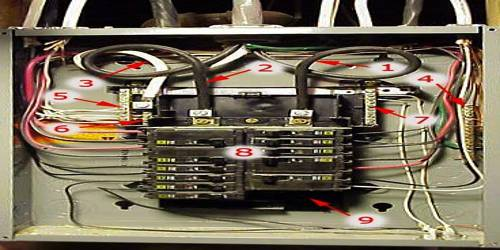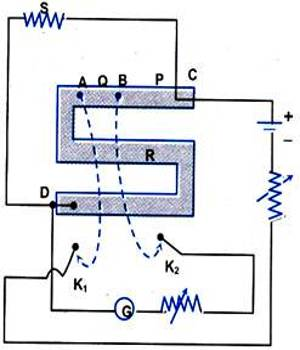# Post Office Box

Post Office Box: A post office box is a practical form of Wheatstone bridge. It is a testing device used to locate faults that may be miles away. The resistances of resistance box that are used as three arms of a Wheatstone and by inserting an unknown resistance in the fourth arm its resistance can be measured by applying Wheatstone bridge principle is called the post office box.

It is an electrical device that is used to find the resistance of the object. It can also compare the resistance of the two wires. Earlier, it was used in the post office to measure the resistance of a wire. So, it is named as post office box. It works on the principle of Wheatstone bridge to identify the resistance of wire connections and then by using wire resistivity and cross-section calculating the length of the wire and thus determining where the cable had broken.

Measurement of unknown resistance using a Post Office Box

A Post Office Box can also be used to measure an unknown resistance. It is a Wheatstone Bridge with three arms P, Q, and R; while the fourth arm(s) is the unknown resistance. P and Q are known as the ratio arms while R is known as the rheostat arm.

At balance, the unknown resistance

S = (P/Q) R                                                           …… (1)

The ratio arms are first adjusted so that they carry 100 W each. The resistance in the rheostat arm is now adjusted so that the galvanometer deflection is in one direction. If R = R0 (ohm) and

R = R0 + 1 (ohm) are the resistance in the rheostat arm, for which the deflection in the galvanometer is in opposite direction, then it implies that the unknown resistance ‘s’ lies between R0 & (R0 + 1) ohm.Now, the resistance in P and Q are made 100 W and 1000 W, respectively, and the process is repeated. Equation (1) is used to compute S. The ratio P/Q is progressively made 1:10, and then 1:100. Thus, resistance S can be accurately measured.

It was used by the engineers in the UK to determine where a break occurred in the cable. A typical post office box is in a wooden box with a hinged lid and a metal or bakelite panel showing circuit connections. Coils of wire are wound non-inductively, mounted in the body of the box, and have a negligible temperature coefficient. Pairs of ratio arms are each 10, 100, 1000 ohms. A resistance arm contains a number of coils from 1 to 5000 ohms with a plug for infinite resistance.